# CBSE Class 12 Mathematics HOTs Matrices and Determinants Set D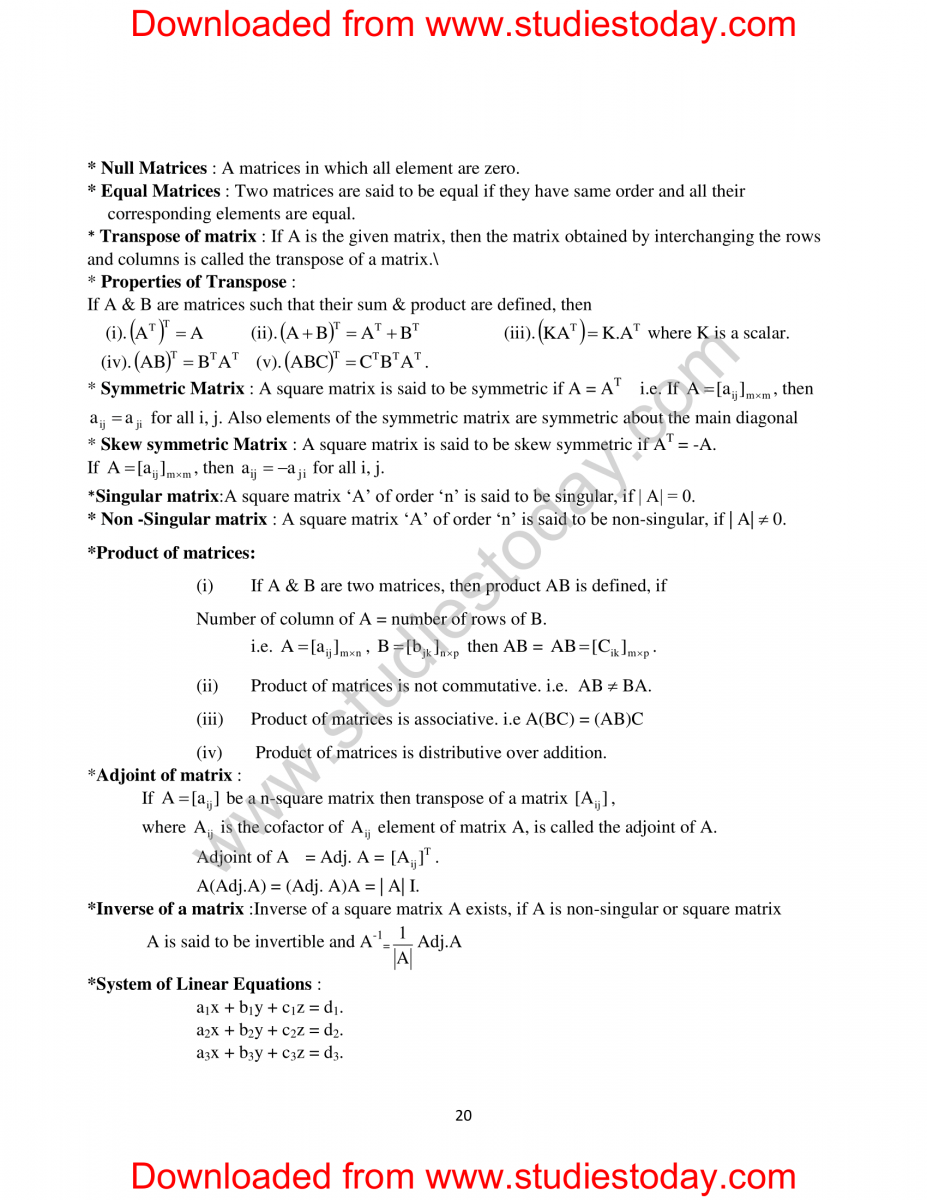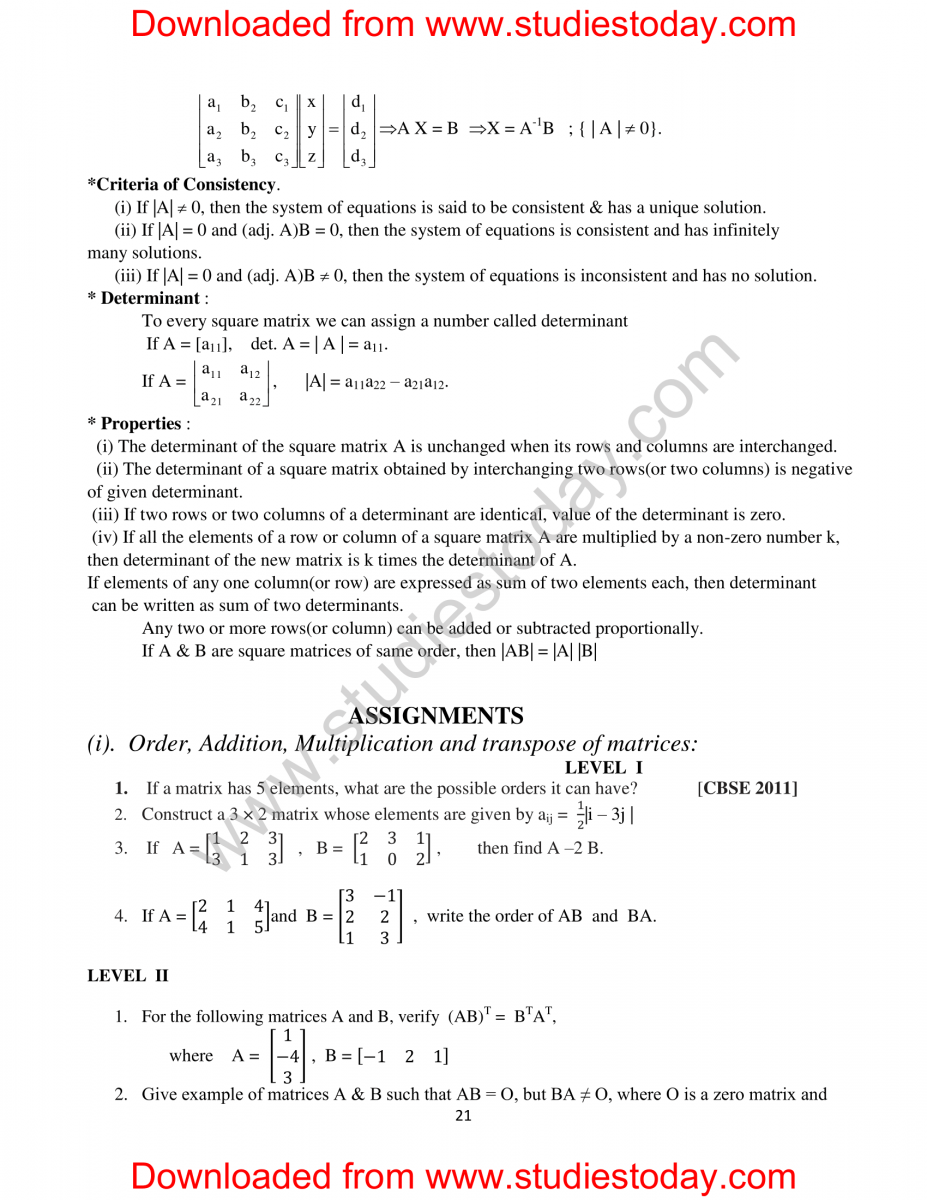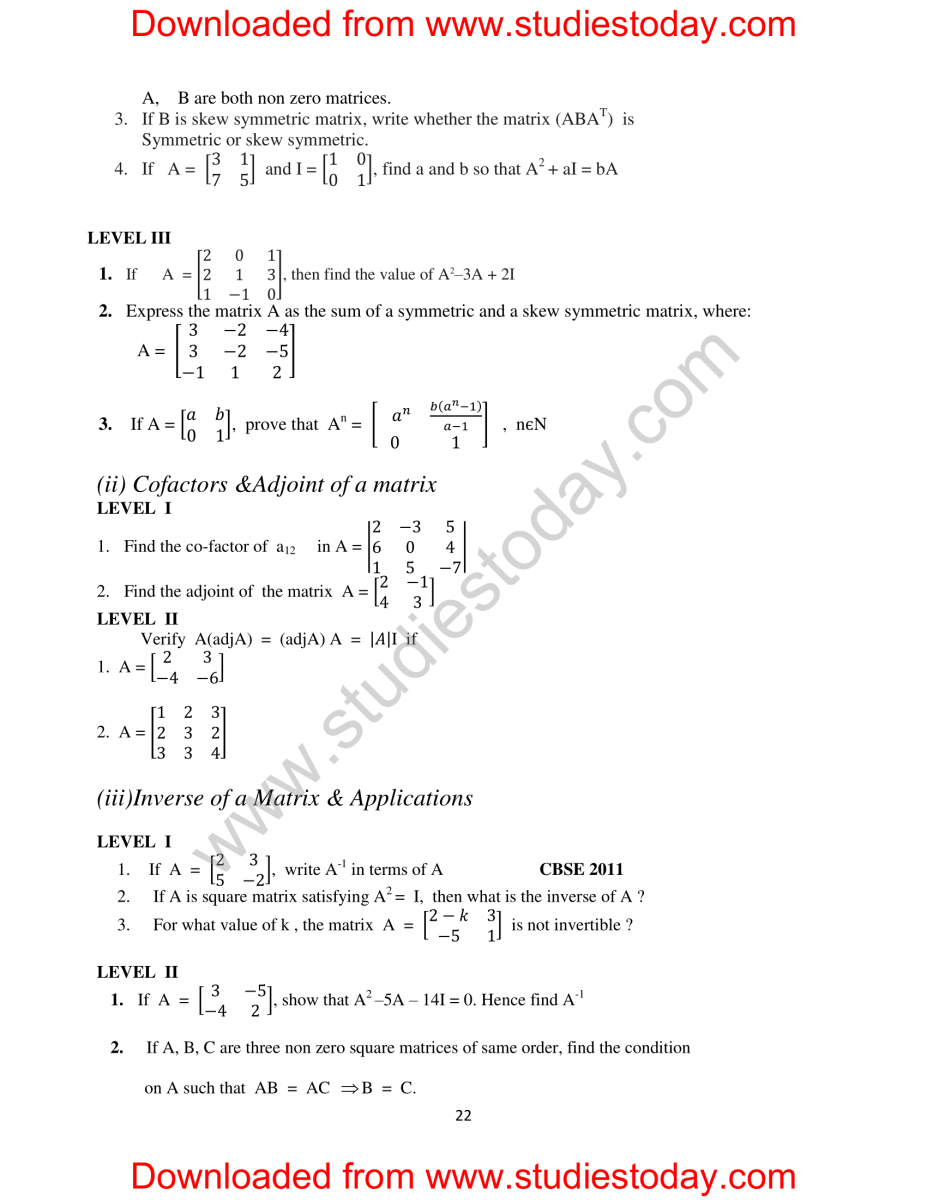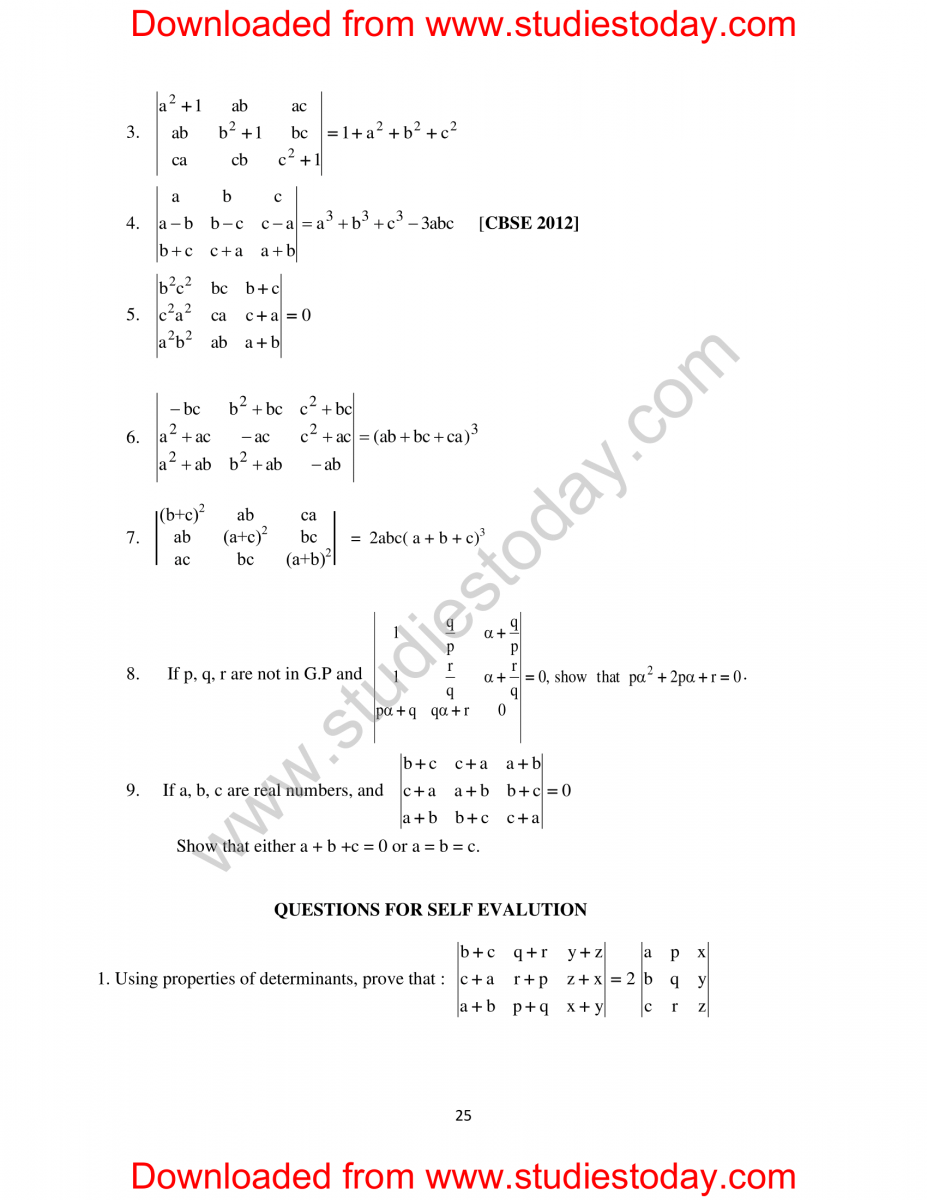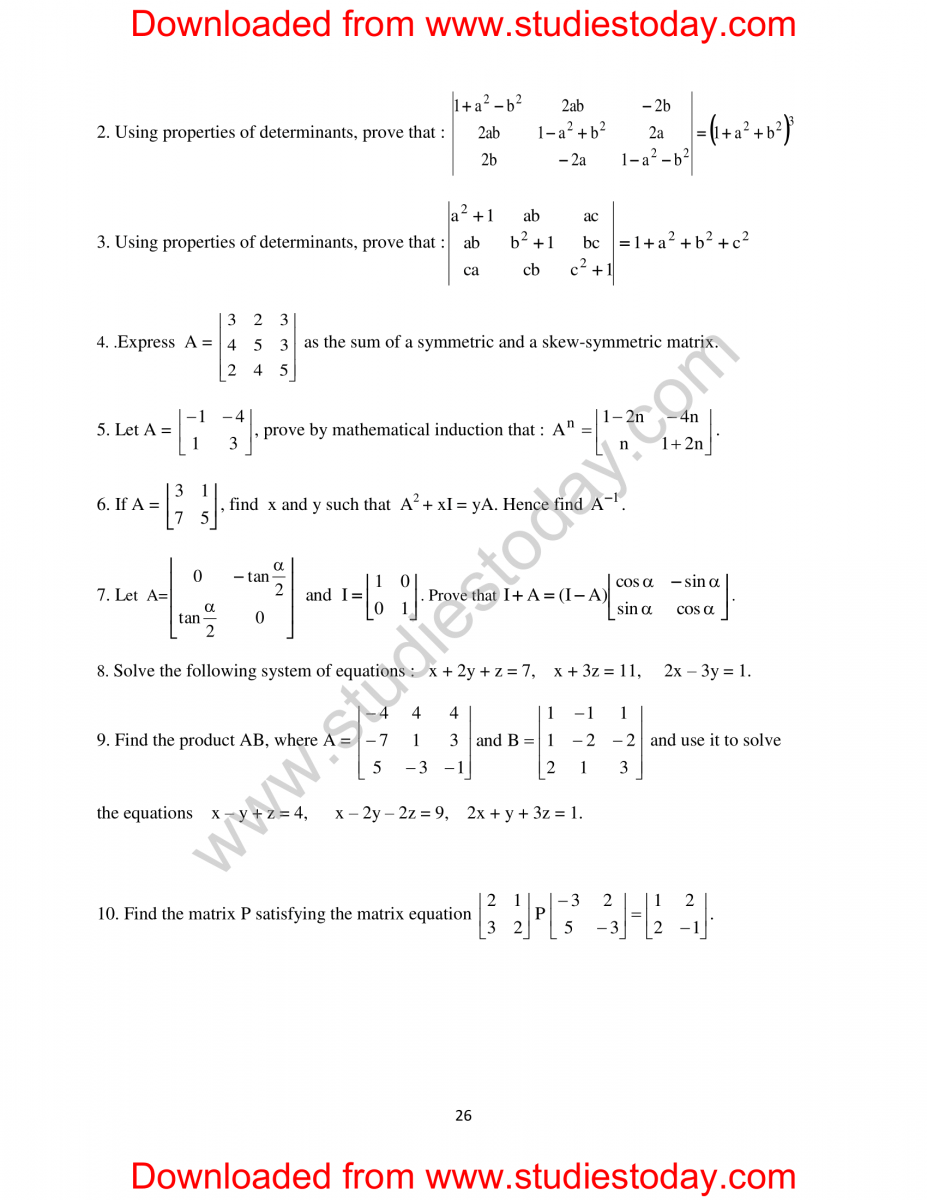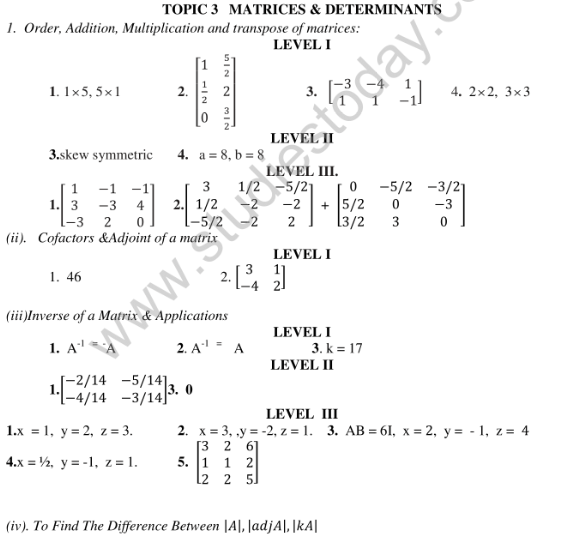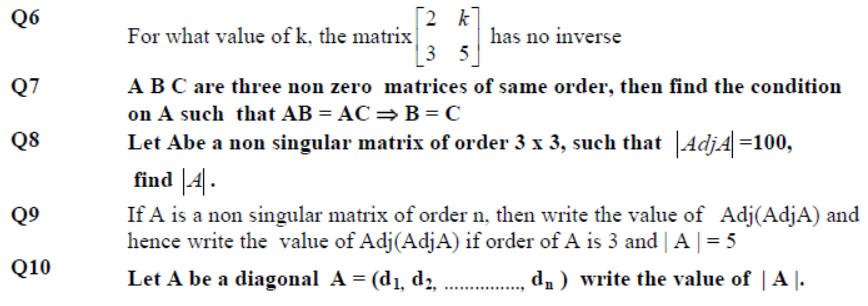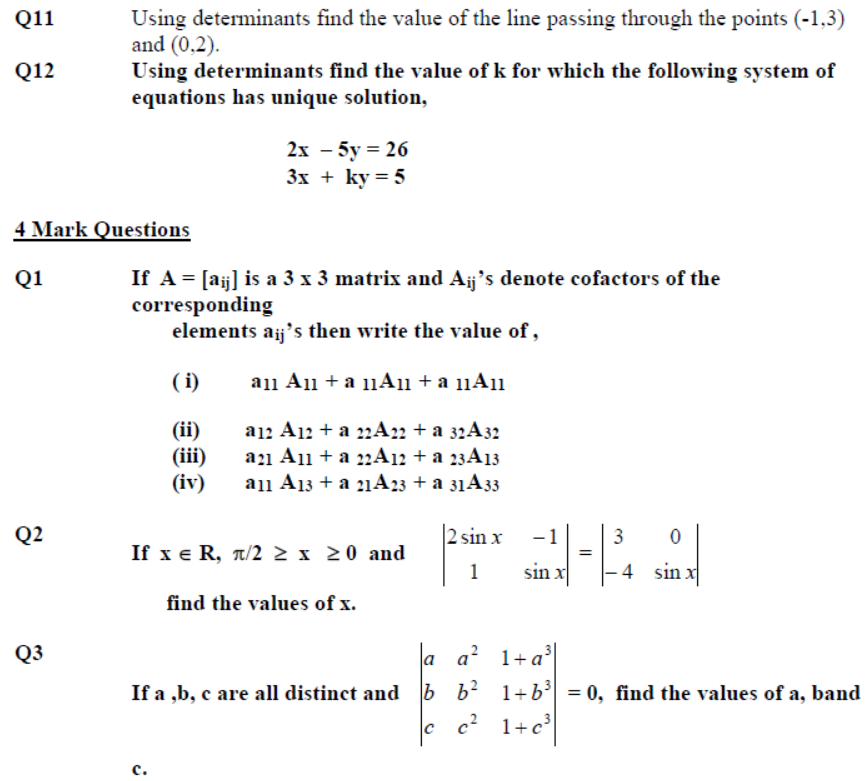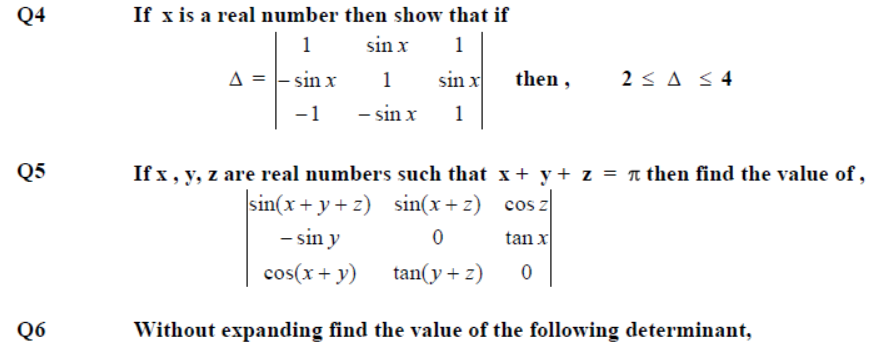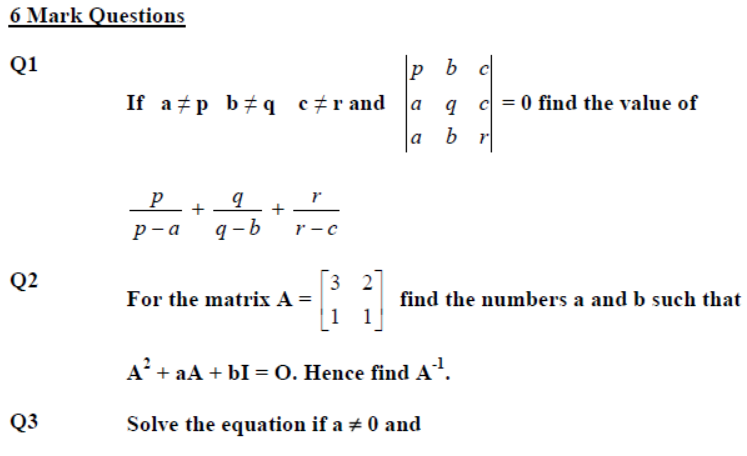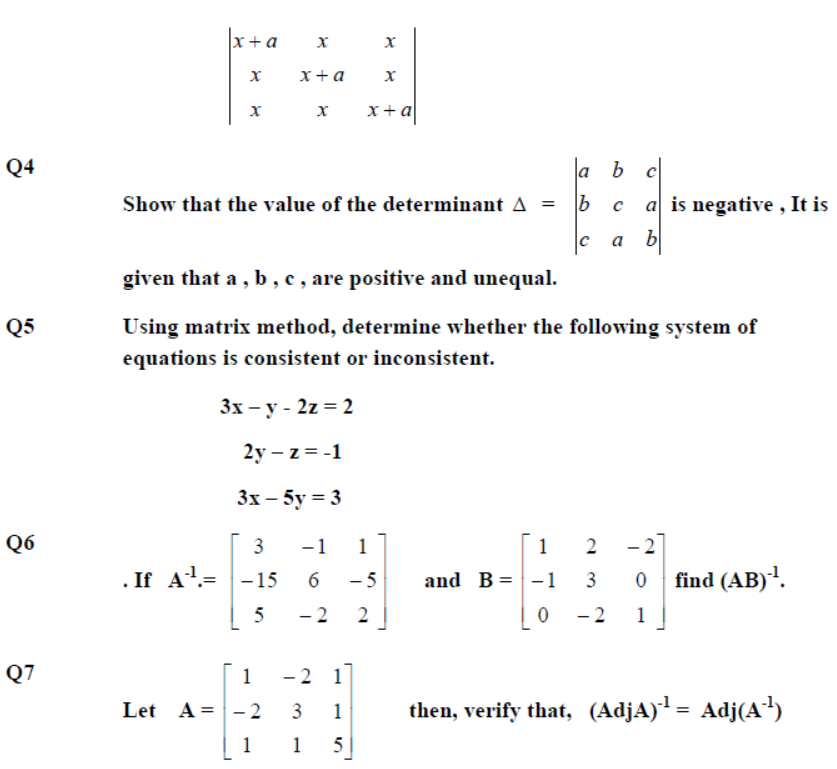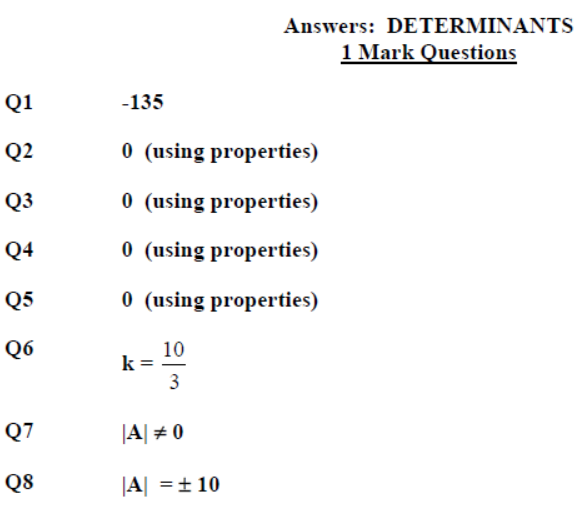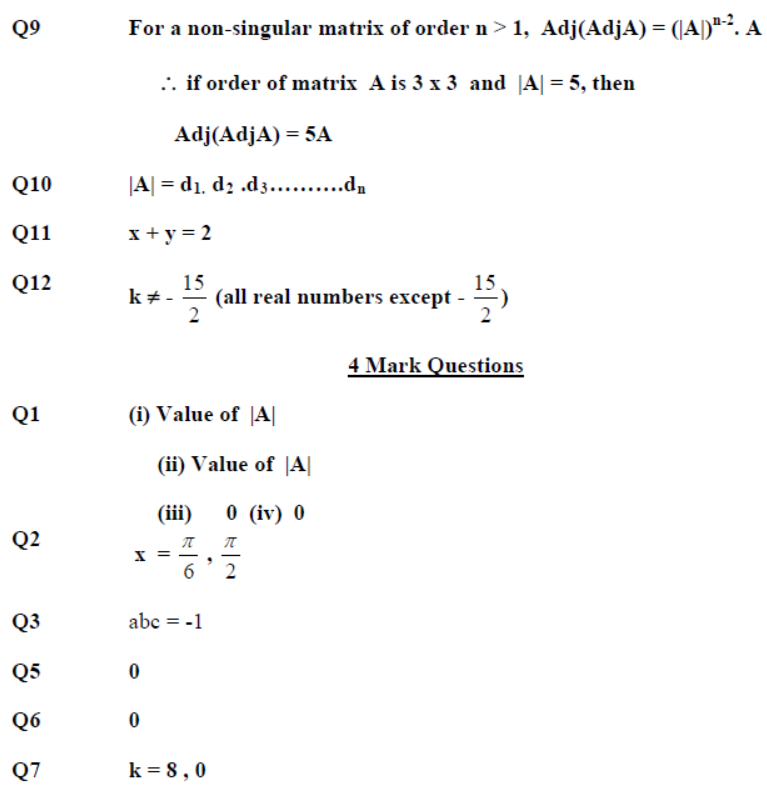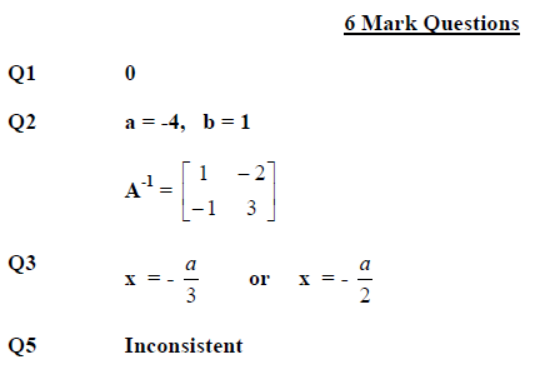## Books recommended by teachers

 CBSE Class 12 Mathematics HOTs Relations and Functions
 CBSE Class 12 Mathematics HOTs Inverse Trigonometric Functions
 CBSE Class 12 Mathematics HOTs Matrices and Determinants
 CBSE Class 12 Mathematics HOTs Matrices and Determinants Set D
 CBSE Class 12 Mathematics HOTs Continuity And Differentiability
 CBSE Class 12 Mathematics HOTs Application of Derivatives
 CBSE Class 12 Mathematics HOTs Application of Integrals
 CBSE Class 12 Mathematics HOTs Differential Equations
 CBSE Class 12 Mathematics HOTs Vectors
 CBSE Class 12 Mathematics HOTs Three Dimensional Geometry
 CBSE Class 12 Mathematics HOTs Linear Programming
 CBSE Class 12 Mathematics HOTs Probability